Get inspired by the success stories of our students in IIT JAM MS, ISI  MStat, CMI MSc DS.  Learn More

# ISI MStat 2018 PSA Problem 11 | Sequence & it's subsequenceThis is a problem from ISI MStat 2018 PSA Problem 11 based on Sequence and subsequence.

## Sequence & it's subsequence - ISI MStat Year 2018 PSA Problem 11

Let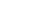be a sequence such that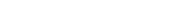Suppose the subsequence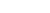is bounded. Then

• (A)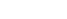is always convergent but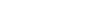need not be convergent.
• (B) bothandare always convergent and have the same limit.
• (C)is not necessarily convergent.
• (D) bothandare always convergent but may have different limits.

### Key Concepts

Sequence

Subsequence

ISI MStat 2018 PSA Problem 11

Introduction to Real Analysis by Bertle Sherbert

## Try with Hints

Given that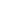is bounded . Again we have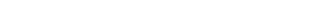which shows that ifis bounded then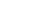is also bounded .

Again bothandboth are monotonic sequence . Hence both converges .

Now we have to see whether they converges to same limit or not ?

As bothandare bounded hence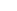is bounded and it's already given that it is monotonic . Henceconverges . So, it's subsequences must converges to same limit . Hence option (B) is correct .

## Subscribe to Cheenta at Youtube

This is a problem from ISI MStat 2018 PSA Problem 11 based on Sequence and subsequence.

## Sequence & it's subsequence - ISI MStat Year 2018 PSA Problem 11

Letbe a sequence such thatSuppose the subsequenceis bounded. Then

• (A)is always convergent butneed not be convergent.
• (B) bothandare always convergent and have the same limit.
• (C)is not necessarily convergent.
• (D) bothandare always convergent but may have different limits.

### Key Concepts

Sequence

Subsequence

ISI MStat 2018 PSA Problem 11

Introduction to Real Analysis by Bertle Sherbert

## Try with Hints

Given thatis bounded . Again we havewhich shows that ifis bounded thenis also bounded .

Again bothandboth are monotonic sequence . Hence both converges .

Now we have to see whether they converges to same limit or not ?

As bothandare bounded henceis bounded and it's already given that it is monotonic . Henceconverges . So, it's subsequences must converges to same limit . Hence option (B) is correct .

## Subscribe to Cheenta at Youtube

This site uses Akismet to reduce spam. Learn how your comment data is processed.

### Knowledge Partner